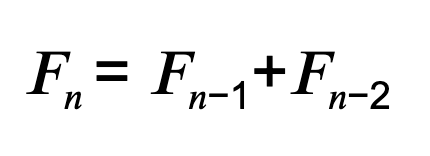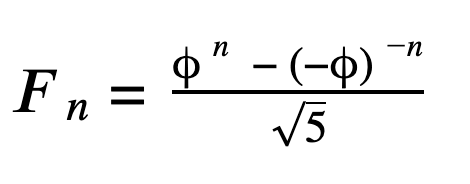Science & Tech

# Fibonacci Sequence Formula: How to Find Fibonacci Numbers

Written by MasterClass

Last updated: Nov 9, 2020 • 4 min read

The Fibonacci sequence is a pattern of numbers that reoccurs throughout nature.Neil deGrasse Tyson Teaches Scientific Thinking and Communication

Renowned astrophysicist Neil deGrasse Tyson teaches you how to find objective truths and shares his tools for communicating what you discover.

## What Is the Fibonacci Sequence?

The Fibonacci sequence is one of the most well-known formulas in number theory and one of the simplest integer sequences defined by a linear recurrence relation. In the Fibonacci sequence of numbers, each number in the sequence is the sum of the two numbers before it, with 0 and 1 as the first two numbers. The Fibonacci series of numbers begins as follows: 0, 1, 1, 2, 3, 5, 8, 13, 21, 34, 55, 89, 144, and so on. Fibonacci's sequence is useful for its applications in advanced mathematics and statistics, computer science, economics, and nature.

## The Origin of the Fibonacci Sequence

The Fibonacci sequence first appears in ancient Sanskrit texts as early as 200 BC, but the sequence wasn't widely known to the western world until 1202 when Italian mathematician Leonardo Pisano Bogollo published it in his book of calculations called Liber Abaci. Leonardo also went by the moniker Leonardo of Pisa, but it wasn't until 1838 that historians gave him the nickname Fibonacci (roughly translating to "son of Bonacci"). In addition to popularizing the Fibonacci sequence, Fibonacci's book Liber Abaci advocated for the use of Hindu-Arabic numerals (1, 2, 3, 4, etc.) and helped replace the Roman numeral system (I, II, III, IV, etc.) throughout Europe.

In Liber Abaci, the Fibonacci sequence was actually used to answer a hypothetical math problem involving rabbit population growth: If a single pair of rabbits mate at the end of every month, then birth a new pair of rabbits one month after they mate, and all new pairs of rabbits follow that same pattern, how many pairs or rabbits will exist in one year? Here's how you'd start answering this problem:

• Begin with 1 pair of rabbits.
• At the end of the first month, there is still only 1 pair of rabbits since they've mated, but have not yet given birth.
• At the end of the second month, there are 2 pairs of rabbits since the first pair have now birthed a second pair.
• At the end of the third month, there are 3 pairs of rabbits. This is because the first pair have birthed a third pair, but the second pair have only mated.
• At the end of the fourth month, there are now 5 pairs of rabbits. This is because the first pair have birthed another pair, and the second pair have now birthed their first pair.

As you can see, this 1, 1, 2, 3, 5 pattern follows the Fibonacci sequence. If you continue for 12 months, the number of pairs will equal 144.

## Fibonacci Number Formula

To calculate each successive Fibonacci number in the Fibonacci series, use the formulawhere 𝐹 is 𝑛th Fibonacci number in the sequence, and the first two numbers, 𝐹0 and 𝐹1 , are set at 0 and 1 respectively.

The only problem with this formula is that it's a recursive formula, meaning it defines each number of the sequence using the preceding numbers. So if you wanted to calculate the tenth number in the Fibonacci sequence, you'd need to first calculate the ninth and eighth, but to get the ninth number you'd need the eighth and seventh, and so on.

To find any number in the Fibonacci sequence without any of the preceding numbers, you can use a closed-form expression called Binet's formula:In Binet's formula, the Greek letter phi (φ) represents an irrational number called the golden ratio: (1 + √ 5)/2, which rounded to the nearest thousandths place equals 1.618.

## Fibonacci Sequence and the Golden Ratio

The golden ratio (or golden section) is an irrational number that results when the ratio of two numbers is the same as the ratio of their sum to the larger of the two numbers. The Fibonacci sequence is closely connected to the golden ratio because as the Fibonacci numbers increase, the ratio of any two consecutive Fibonacci numbers gets closer and closer to the golden ratio.

### MasterClass

#### Suggested for You

Online classes taught by the world’s greatest minds. Extend your knowledge in these categories.

## Fibonacci Sequence in Nature

### Think Like a ProRenowned astrophysicist Neil deGrasse Tyson teaches you how to find objective truths and shares his tools for communicating what you discover.

View Class

There's considerable misinformation about where you may find the Fibonacci sequence and golden ratio in the real world; despite what you may read, the golden ratio was not used to build the pyramids at Giza, and the nautilus seashell does not grow new cells based on the Fibonacci sequence.

But these mathematical properties behind the Fibonacci sequence and golden ratio appear throughout nature in a number of ways. For example, you can find the golden ratio in the spiral arrangement of leaves (called a phyllotaxis) on some plants, or in the golden spiral pattern of pinecones, cauliflower, pineapples, and the arrangement of seeds in sunflowers. Additionally, the number of petals on a flower is typically a Fibonacci number.

Further, a honeybee drone's family tree follows the Fibonacci sequence. This is because a male drone hatches from an unfertilized egg and only has one parent, while female bees have two parents. This results in a drone’s family tree consisting of one parent, two grandparents, three great-grandparents, five great-great-grandparents, and so on throughout the Fibonacci sequence.